Home > A2C > Chapter 8 > Lesson 8.1.7 > Problem8-105

8-105.
1. Evaluate each of the following trig expressions without using a calculator. Homework Help ✎

1. sin(180°)

2. sin(360°)

3. sin(−90°)

4. sin(510°)

5. cos(90°)

6. tan(−90°)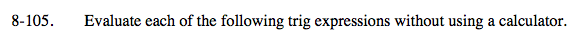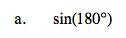Every point on the unit circle is defined as (cos θ, sin θ).
Where is 180° on the unit circle?
Where is the y-value at that point?

0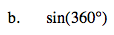See part (a).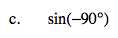When an angle is negative, it opens clockwise from 0° on the unit circle.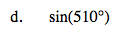Find an equivalent angle between 0° and 360° by subtracting 510 − 360. Notice that the reference
triangle is a 30°-60°-90° triangle. Find the sin 30°.

0.5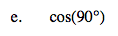What is the x-value in this location?

0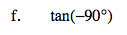$\text{Remember the tangent is the ratio of } \frac{y}{x}.$

undefined